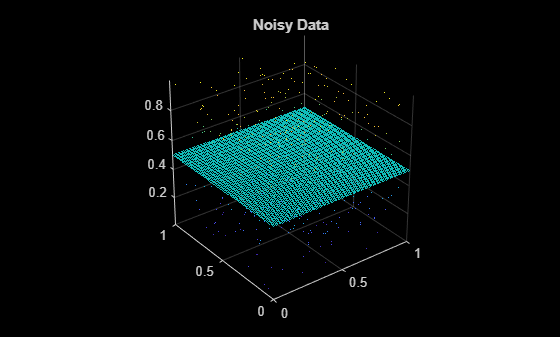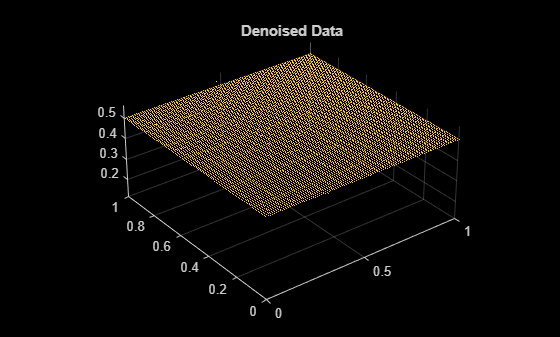# pcdenoise

Remove noise from 3-D point cloud

## Syntax

``ptCloudOut = pcdenoise(ptCloudIn)``
``````[ptCloudOut,inlierIndices,outlierIndices] = pcdenoise(ptCloudIn) ``````
``````[ptCloudOut,___] = pcdenoise(___Name,Value)``````

## Description

example

````ptCloudOut = pcdenoise(ptCloudIn)` returns a filtered point cloud that removes outliers.```
``````[ptCloudOut,inlierIndices,outlierIndices] = pcdenoise(ptCloudIn) ``` additionally returns the linear indices to the points that are identified as inliers and outliers.```
``````[ptCloudOut,___] = pcdenoise(___Name,Value)``` uses additional options specified by one or more `Name,Value` pair arguments, using any of the preceding syntaxes.```

## Examples

collapse all

Create a plane point cloud.

```gv = 0:0.01:1; [X,Y] = meshgrid(gv,gv); ptCloud = pointCloud([X(:),Y(:),0.5*ones(numel(X),1)]); figure pcshow(ptCloud); title('Original Data');``````noise = rand(500, 3); ptCloudA = pointCloud([ptCloud.Location; noise]); figure pcshow(ptCloudA); title('Noisy Data');```Remove outliers.

```ptCloudB = pcdenoise(ptCloudA); figure; pcshow(ptCloudB); title('Denoised Data');```## Input Arguments

collapse all

Point cloud, specified as a `pointCloud` object.

### Name-Value Pair Arguments

Specify optional comma-separated pairs of `Name,Value` arguments. `Name` is the argument name and `Value` is the corresponding value. `Name` must appear inside quotes. You can specify several name and value pair arguments in any order as `Name1,Value1,...,NameN,ValueN`.

Example: `'Threshold'`,`1.0` sets the threshold to `1.0`.

Number of nearest neighbor points, specified as the comma-separated pair consisting of '`NumNeighbors`' and a positive integer in pixels. The value is used to estimate the mean of the average distance to neighbors of all points. Decreasing this value makes the filter more sensitive to noise. Increasing this value increases the number of computations.

Data Types: `single` | `double`

Outlier threshold, specified as the comma-separated pair consisting of '`Threshold`' and a scalar. By default, the threshold is one standard deviation from the mean of the average distance to neighbors of all points. A point is considered to be an outlier if the average distance to its k-nearest neighbors is above the specified threshold.

Data Types: `single` | `double`

## Output Arguments

collapse all

Filtered point cloud, returned as a `pointCloud` object.

Linear index of inlier points, returned as a 1-by-N vector.

Data Types: `uint32`

Linear index of outlier points, returned as a 1-by-N vector of linear indices.

Data Types: `uint32`

 Rusu, R. B., Z. C. Marton, N. Blodow, M. Dolha, and M. Beetz. “Towards 3D Point Cloud Based Object Maps for Household Environments”. Robotics and Autonomous Systems Journal. 2008.